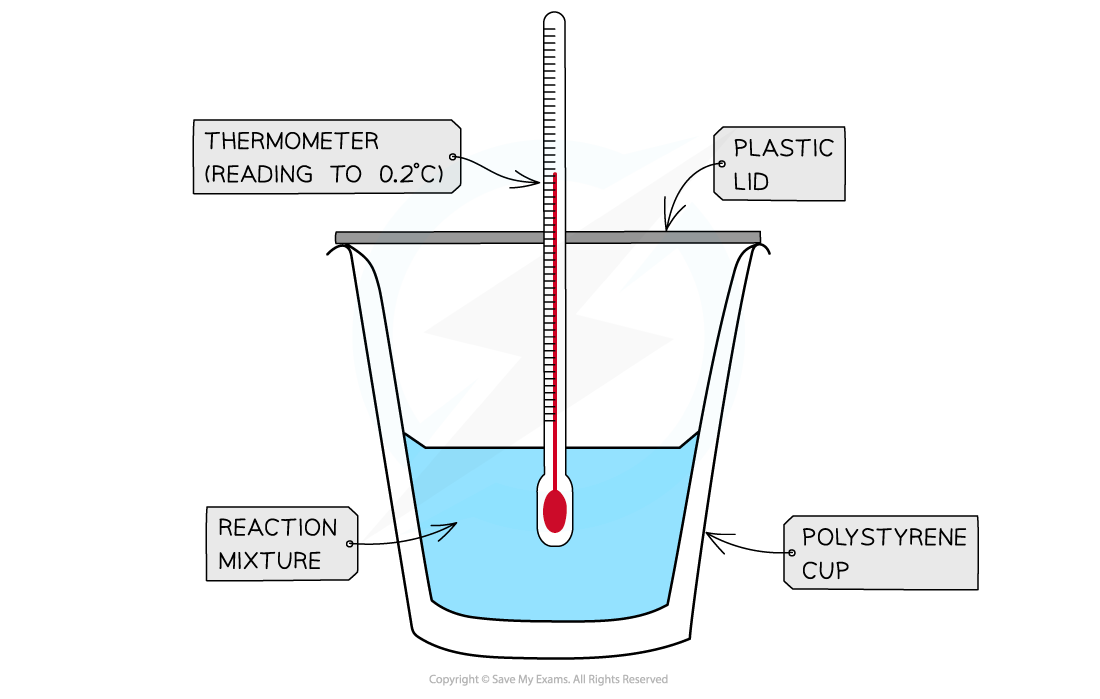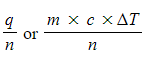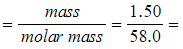# Edexcel A Level Chemistry:复习笔记1.8.3 Calorimetry

### Calculating Energy Transferred, Q

#### Measuring enthalpy changes

• Calorimetry is the measurement enthalpy changes in chemical reactions
• A simple calorimeter can be made from a polystyrene drinking cup, a vacuum flask or metal canA polystyrene cup can act as a calorimeter to find enthalpy changes in a chemical reaction

• The energy needed to increase the temperature of 1 g of a substance by 1 oC is called the specific heat capacity (c ) of the liquid
• The specific heat capacity of water is 4.18 J g-1 K-1
• The energy transferred as heat can be calculated by:Equation for calculating energy transferred in a calorimeter

#### Worked Example

Specific heat capacity calculations

In a calorimetry experiment 2.50 g of methane is burnt in excess oxygen.

30% of the energy released during the combustion is absorbed by 500 g of water, the temperature of which rises from 25 °C to 68 °C.

The specific heat capacity of water is 4.18 J g-1 K−1

What is the total energy released per gram of methane burnt?

Step 1

• q = m x c x ΔT
• m (of water) = 500 g
• c (of water) = 4.18 J g-1 °C-1
• ΔT (of water) = 68 oC - 25 oC = 43 oC

Step 2:

• q = 500 x 4.18 x 43 = 89 870 J

Step 3:

• This is only 30% of the total energy released by methane
• Total energy x 0.3 = 89 870 J
• Total energy = 299 567 J

Step 4:

• This is released by 2.50 g of methane
• Energy released by 1.00 g of methane = 299 567 ÷ 2.50 = 120 000 J g-1 (to 3 s.f.) or 120 kJ g-1

### Calculating Enthalpy Changes

• Aqueous solutions of acid, alkalis and salts are assumed to be largely water so you can just use the m and c values of water when calculating the energy transferred.
• To calculate any changes in enthalpy per mole of a reactant or product the following relationship can be used:

ΔH =• When there is a rise in temperature, the value for ΔH becomes negative suggesting that the reaction is exothermic
• This means that your value should be negative for an exothermic reaction, e.g. combustion
• When the temperature falls, the value for ΔH becomes positive suggesting that the reaction is endothermic
• This means that your value should be positive for an endothermic reaction, e.g. combustion

#### Worked Example

1.50 g of an organic liquid (Mr = 58.0) underwent complete combustion. The heat formed raised the temperature of 100 g of water from 20 oC to 75 oC.

Calculate the enthalpy of combustion for the organic liquid

Step 1: Calculate the energy released by the organic liquid

• Q = mcΔT
• Q = 100 x 4.18 x (75 - 20)
• Q = 22990 J
• Q = 22.99 kJ

Step 2: Calculate the number of moles of the organic liquid

• Number of moles =0.0259 moles (to 3s.f.)

Step 3: Calculate the enthalpy change of combustion

• ΔcHθ-887 kJ mol-1 (to 3s.f.)
• Remember, combustion is an exothermic process and will, therefore, be a negative enthalpy change value